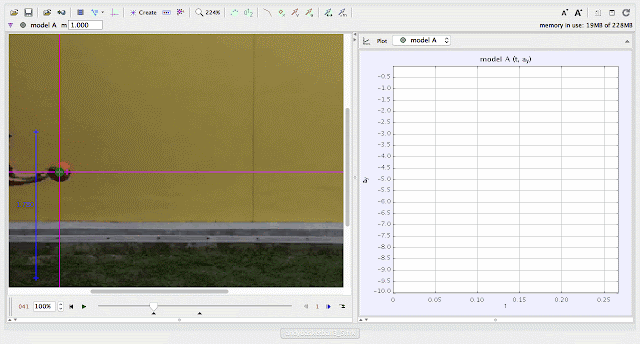{source}
<?phprequire_once JPATH_SITE.'/TTcustom/TT_contentparser.php';//include 'TT_contentparser.php';$$parameters = array( "topicname" => "02_newtonianmechanics_2kinematics", //e.g. 02_newtonianmechanics_2kinematics"modelname" => "andycar4_5"); //e.g. airpuckconstantvelocity.trzecho generateSimHTML($$parameters, "TRZ");
?>
{/source}

### ICT Connection Lesson

http://library.opal.moe.edu.sg/ictc&func=view&rid=2094using Tracker Model Builder to create theoretic graphs for simplifying Physics concepts. uniform acceleration motion is illustrated through position x versus time graphusing Tracker Model Builder to create theoretic graphs for simplifying Physics concepts. uniformly increasing velocity motion is illustrated through position vx versus time graphusing Tracker Model Builder to create theoretic graphs for simplifying Physics concepts. uniform acceleration motion is illustrated through position ax versus time graph

end faq

Rating 5.00 (1 Vote)